Note

# What is Margin Level?

· Reading 62

What does “Margin Level” mean?

The Margin Level is the percentage (%) value based on the amount of Equity versus Used Margin.

Margin Level allows you to know how much of your funds are available for new trades.

The higher the Margin Level, the more Free Margin you have available to trade.

The lower the Margin Level, the less Free Margin available to trade, which could result in something very bad…like a Margin Call or a Stop Out (which will be discussed later).

## How to Calculate Margin Level

Here’s how to calculate Margin Level:

```Margin Level = (Equity / Used Margin) x 100%
```

Your trading platform will automatically calculate and display your Margin Level.

If you don’t have any trades open, your Margin Level will be ZERO.

Margin Level is very important. Forex brokers use margin levels to determine whether you can open additional positions.Different brokers set different Margin Level limits, but most brokers set this limit at 100%.

This means that when your Equity is equal or less than your Used Margin, you will NOT be able to open any new positions.

If you want to open new positions, you will have to close existing positions first.

## Example #1: Open a long USD/JPY position with 1 mini lot

Let’s say you have an account balance of \$1,000.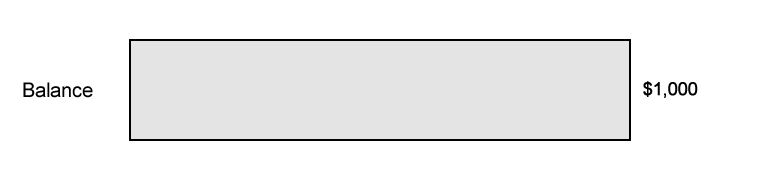### Step 1: Calculate Required Margin

You want to go long USD/JPY and want to open 1 mini lot (10,000 units) position. The Margin Requirement is 4%.

How much margin (Required Margin) will you need to open the position?

Since USD is the base currency. this mini lot is 10,000 dollars, which means the position’s Notional Value is \$10,000.

```Required Margin = Notional Value x Margin Requirement
\$400 = \$10,000 x .04
```

Assuming your trading account is denominated in USD, since the Margin Requirement is 4%, the Required Margin will be \$400.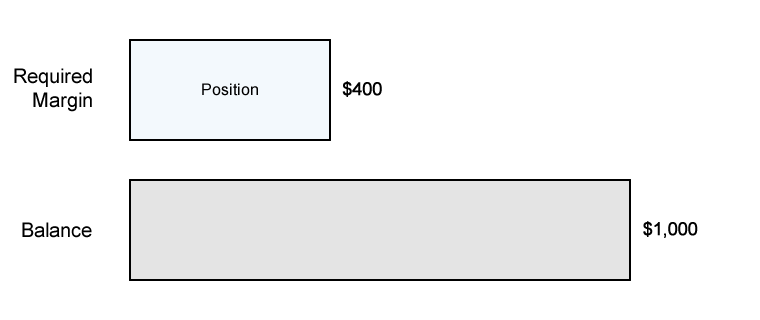### Step 2: Calculate Used Margin

Aside from the trade we just entered, there aren’t any other trades open.

Since we just have a single position open, the Used Margin will be the same as Required Margin.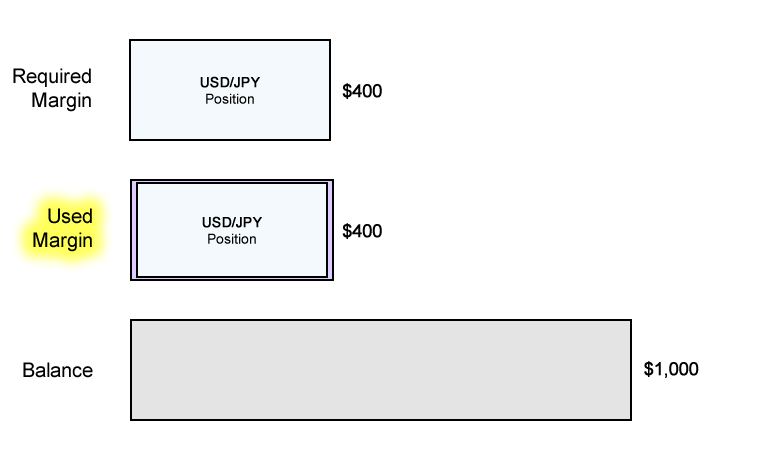### Step 3: Calculate Equity

Let’s assume that the price has moved slightly in your favor and your position is now trading at breakeven.

This means that your Floating P/L is \$0.

Let’s calculate the Equity:

```Equity = Account Balance + Floating Profits (or Losses)
\$1,000 = \$1,000 + \$0
```

The Equity in your account is now \$1,000.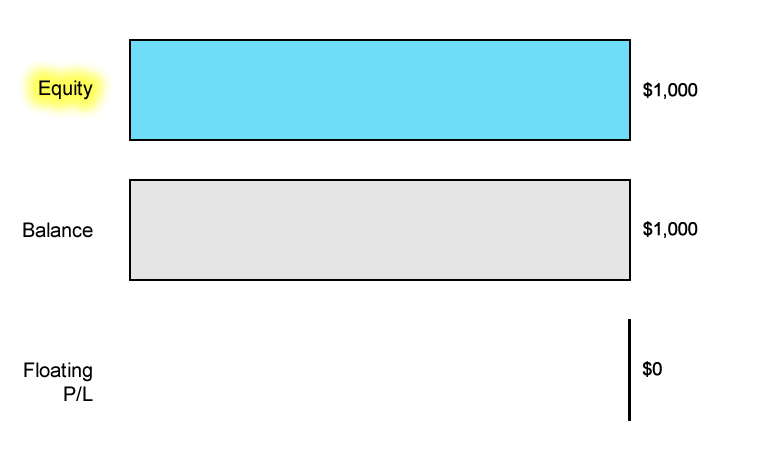### Step 4: Calculate Margin Level

Now that we know the Equity, we can now calculate the Margin Level:

```Margin Level = (Equity / Used Margin) x 100%
250% = (\$1,000 / \$400) x 100%
```

The Margin Level is 250%.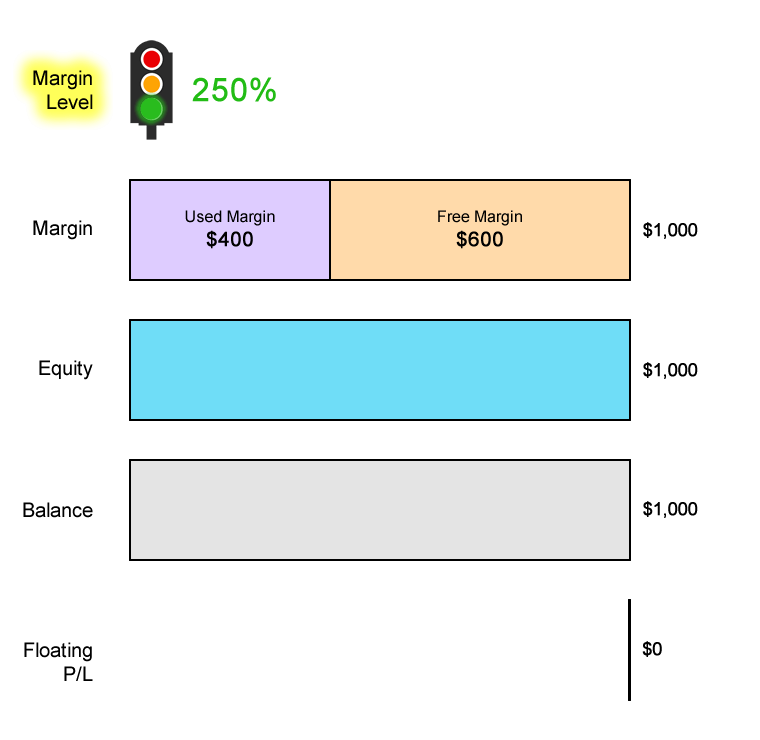If the Margin Level is 100% or less, most trading platforms will not allow you to open new trades.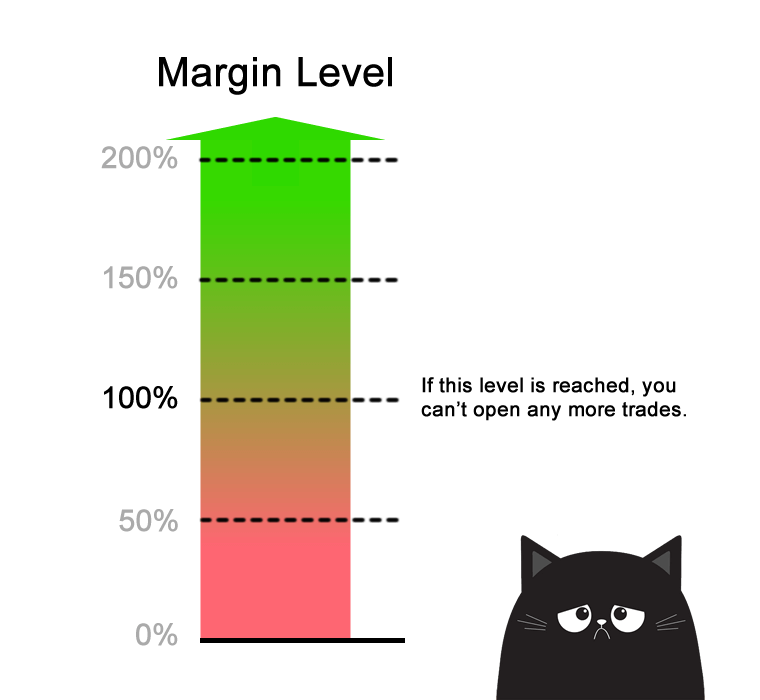In the example, since your current Margin Level is 250%, which is way above 100%, you’ll still be able to open new trades.

As long as the Margin Level is above 100%, then your account has the “green light” to continue to open new trades.

## Recap

In this lesson, we learned about the following:

• Margin Level is the ratio between Equity and Used Margin. It is expressed as a percentage (%).
• For example, if your Equity is \$5,000 and the Used Margin is \$1,000, the Margin Level is 500%.

Reprinted from Babypips，the copyright all reserved by the original author.

Disclaimer: The content above represents only the views of the author or guest. It does not represent any views or positions of FOLLOWME and does not mean that FOLLOWME agrees with its statement or description, nor does it constitute any investment advice. For all actions taken by visitors based on information provided by the FOLLOWME community, the community does not assume any form of liability unless otherwise expressly promised in writing.

If you like, reward to support.## HotNo older comments, be the first to grab the sofa.